# Exam-Style Questions on Functions

## Problems on Functions adapted from questions set in previous Mathematics exams.

### 1.

GCSE Higher

$$f(x) = \frac{2x}{5} + 7$$ and $$g(x) = 10x^2 - 15$$ for all values of $$x$$.

Find $$fg(x)$$.

Give your answer in the form $$ax^2 + b$$ where $$a$$ and $$b$$ are integers.

### 2.

GCSE Higher

The function $$f$$ is described by the following formula:

$$f(x) = 3x^2 - 2x^3$$

Calculate the value of $$f(-5)$$

### 3.

IGCSE Extended

The table shows some values (rounded to one decimal place) for the function $$y=\frac{2}{x^2}-x, x\neq 0$$.

 $$x$$ $$y$$ -3 -2 -1 -0.5 0.5 1 2 3 4 3.2 2.5 8.5 7.5 1 -2.8

(a) Complete the table of values.

(b) Draw the graph of $$y=\frac{2}{x^2}-x$$ for $$-3\le x \le -0.5$$ and $$0.5\le x\le 4$$.

(c) Use your graph to solve the equation $$\frac{2}{x^2}-x-3=0$$

(d) Use your graph to solve the equation $$\frac{2}{x^2}-x=1-2x$$

(e) By drawing a suitable tangent, find an estimate of the gradient of the curve at the point where x = 1.

(f) Using algebra, show that you can use the graph at $$y=0$$ to find $$\sqrt2$$

### 4.

GCSE Higher

(a) A function is represented by the following function machine.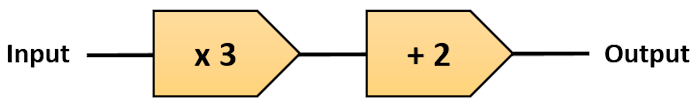A number is input into the machine and the output is used as a new input.

If the second output is 53 work out the number that was the first input.

(b) A number is input into the machine and the output produced is the same number. Work out what this number could have been.

(c) Another function machine is shown below.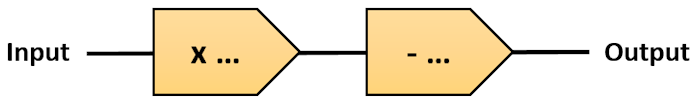If the Input is 2, the Output is 7.
If the Input is 6, the Output is 27.
Use this information to fill in the two boxes.

### 5.

IGCSE Extended

If $$f(x)=5-4x$$ and $$g(x)=4^{-x}$$ then:

(a) Find $$f(3x)$$ in terms of $$x$$.

(b) Find $$ff(x)$$ in its simplest form.

(c) Work out $$gg(–1)$$ give your answer as a fraction.

(d) Find $$f^{–1}(x)$$, the inverse of $$f(x)$$.

(e) Solve the equation $$gf(x)= 1$$.

### 6.

GCSE Higher

Here is a function machine that produces two outputs, A and B.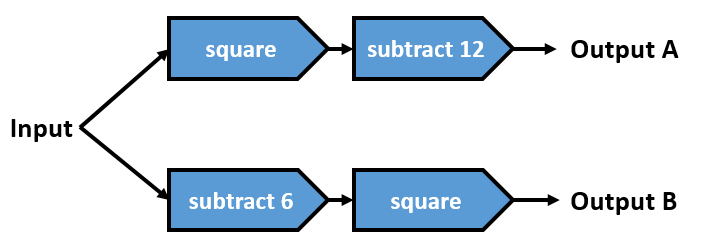Work out the range of input values for which the output A is less than the output B.

### 7.

IB Standard

Consider the graph of $$f(x)=a\sin(b(x+c))+12$$, for $$0\le x\le 24$$.

The graph has a maximum at (8, 22) and a minimum at (18, 2).

(a) Find the value of $$a$$.

(b) Find the value of $$b$$.

(c) Find the value of $$c$$.

(d) Solve $$f(x)=5$$.

### 8.

IB Standard

Let $$f (x)=a(x-b)^2+c$$. The vertex of the graph of $$f$$ is at (4, -3) and the graph passes through (3, 2).

(a) Find the value of $$c$$.

(b) Find the value of $$b$$.

(c) Find the value of $$a$$.

### 9.

IB Studies

The circumference of a given circle $$C$$ can be represented by the function $$C(A) =2\sqrt{A \pi}, A \ge 0$$ where $$A$$ is the area of the circle. The graph of the function $$C$$ is shown for $$0 \le A \le 16$$.(a) Use the graph to find the value of $$C(8)$$ to the nearest whole number.

(b) The range of $$C(A)$$ is $$0 \le C(A) \le n$$. Write down the value of $$n$$.

(c) On the axes above, draw the graph of the inverse function, $$C^{-1}$$.

(d) In the context of the question, explain the meaning of $$C^{-1}(12)$$.

### 10.

IB StandardThe diagram shows the graph of $$y=f(x)$$, for $$-3\le x \le 4$$.

The graph passes through the point (4,0.65).

(a) Find the value of $$f(-2)$$;

(b) Find the value of $$f^{-1}(0)$$;

(c) Find the domain of $$f^{-1}$$.

(d) Sketch the graph of $$f^{-1}$$.

### 11.

IB Standard

The diagram shows part of the graph of $$y=asinbx+c$$ with a minimum at $$(-2.5,-2)$$ and a maximum at $$(2.5,4)$$.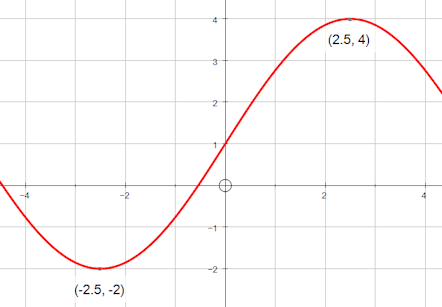(a) Find $$a$$.

(b) Find $$b$$.

(c) Find $$c$$.

### 12.

IB Standard

The Big Wheel at Fantasy Fun Fayre rotates clockwise at a constant speed completing 15 rotations every hour. The wheel has a diameter of 90 metres and the bottom of the wheel is 6 metres above the ground.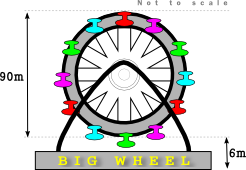A cabin starts at the bottom of the wheel with the top of the cabin 6m above the ground.

(a) Find the greatest height of the top of the cabin reaches as the wheel rotates.

After $$t$$ minutes, the height $$h(t)$$ metres above the ground of the top of a cabin is given by the function $$h(t)=51-a\cos bt$$.

(b) Find the period of $$h(t)$$

(c) Find the value of $$b$$.

(d) Find the value of $$a$$.

(e) Sketch the graph of $$h(t)$$ , for $$0\le t\le 5$$.

(f) In one rotation of the wheel, find the probability that a randomly selected seat is at least 70 metres above the ground. Give your answer to two decimal places.

### 13.

IB Standard

The population of sheep on a ranch is modelled by the function $$P(t)= 65 \sin(0.4t-3.2)+450$$, where t is in months, and $$t=1$$ corresponds to 1st January 2015.

(a) Find the number of sheep on the ranch on 1st July 2015.

(b) Find the rate of change of the sheep population on 1st July 2015.

(c) Explain your answer to part (b) with reference to the sheep population size on 1st July2015.

### 14.

IB Standard

Let $$f(x)=5x^2-20x+k$$. The equation $$f(x)=0$$ has two equal roots.

(a) Write down the value of the discriminant.

(b) Hence, show that $$k=20$$.

The graph of $$f$$ has its vertex on the x-axis.

(c) Find the coordinates of the vertex of the graph of $$f$$.

(d) Write down the solution of $$f(x)=0$$.

The function can be written in the form $$f(x)=a(x-h)^2+j$$.

(e) Find the value of $$a$$.

(f) Find the value of $$h$$.

(g) Find the value of $$j$$.

(h) The graph of a function $$g$$ is obtained from the graph of $$f$$ by a reflection in the x-axis, followed by a translation by the vector $$\begin{pmatrix} 0 \\ 3 \\ \end{pmatrix}$$. Find $$g$$, giving your answer in the form $$g(x)=Ax^2+Bx+C$$.

### 15.

The functions f and g are defined as follows:

$$f(x) = \frac{x+4}{5}$$ $$g(x) = 10x - 3$$

(a) Find $$(g \circ f)(x)$$

(b) Given that $$(g \circ f)^{-1} (a) = 5$$ , find the value of $$a$$.

### 16.

IB Standard

The diagram shows part of the graph of $$f(x)=Ae^{kx}+2$$.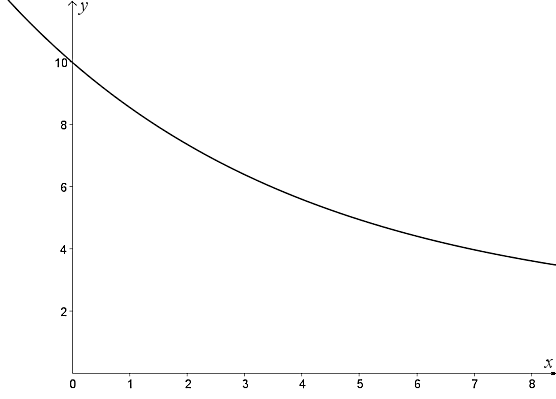The y-intercept is at $$(0,10)$$.

(a) Show that $$A=8$$.

(b) Given that $$f(8)=3.62$$ (correct to 3 significant figures), find the value of $$k$$.

(c) (i) Using your value of $$k$$, find $$f'(x)$$.

(ii) Hence, explain why $$f$$ is a decreasing function.

(iii) Find the equation of the horizontal asymptote of the graph $$f$$.

Let $$g(x)=-x^2+7x+5$$

(d) Find the area enclosed by the graphs of $$f$$ and $$g$$.

### 17.

IB Standard

Part of the graph of $$f(x) = {\log _b}(x + 4)$$ for $$x > - 4$$ is shown below.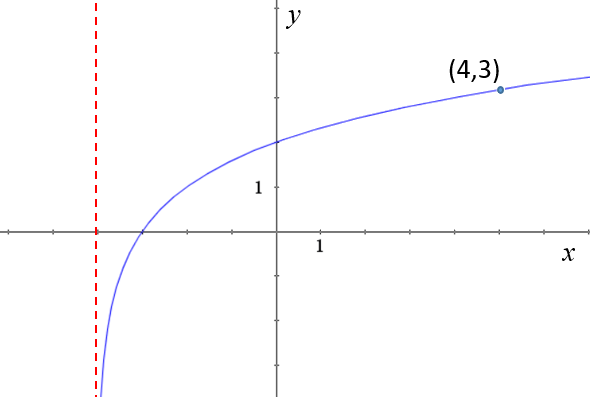The graph passes through A(4, 3) , has an x-intercept at (-3, 0) and has an asymptote at $$x = - 4$$.

(a) Find the value of $$b$$.

The graph of $$f(x)$$ is reflected in the line $$y = x$$ to give the graph of $$g(x)$$.

(b) Write down the y-intercept of the graph of $$g(x)$$.

(c) Sketch the graph of $$g(x)$$, noting clearly any asymptotes and the image of A.

(d) Find $$g(x)$$ in terms of $$x$$.

### 18.

A-Level

The functions $$f$$ and $$g$$ are defined as:

$$f(x) = \sqrt{3x+4} \text{ for } x \ge -1$$ $$g(x) = x^2 - 3x \text{ for all real values of }x$$

(a) Find $$f^{-1}(x)$$

(b) State the domain of $$f^{-1}$$.

(c) Find the range of $$g$$.

(d) Find $$gf(x)$$.

(e) Solve the equation $$gf(x) = 10$$.

### 19.

IB Standard

Let $$f(x)=\sin ( \frac {\pi}{4}x) + \cos ( \frac {\pi}{4}x)$$, for $$-4\le x \le 4$$

(a) Sketch the graph of $$f$$.

(b) Find the values of $$x$$ where the function is decreasing.

(c) The function $$f$$ can also be written in the form $$f(x)=a\sin ( \frac {\pi}{4}(x+c))$$ where $$a\in \mathbf R$$ and $$0 \le c \le 2$$. Find the value of $$a$$ and $$c$$.

### 20.

A-Level

The function $$f$$ is defined as $$f(x) = 12x^3 - 5x^2 -11x + 6$$.

(a) Use the Factor Theorem to show that $$(4x-3)$$ is a factor of $$f(x)$$

(b) Express $$f(x)$$ as a product of linear factors.

(c) The function $$g$$ is defined as $$g( \theta )= 6 \cos \theta \cos 2\theta + 5 \sin^2 \theta - 5 \cos \theta + 1$$. Show that the function $$g( \theta )$$ can be written as $$f(x)$$, where $$x = \cos \theta$$.

(d) Hence solve the equation $$g(\theta ) = 0$$, giving your answers, in radians, in the interval $$0 \le \theta \le 2 \pi$$.

### 21.

Consider a function $$f$$, such that $$f(x)=7.2\sin(\frac{\pi}{6}x + 2) + b$$ where $$0\le x \le 12$$

(a) Find the period of $$f$$.

The function f has a local maximum at the point (11.18,10.3) , and a local minimum at (5.18.-4.1).

(b) Find the value of b.

(c) Hence, find the value of $$f(7)$$.

A second function $$g$$ is given by $$g(x)=a\sin(\frac{2\pi}{7}(x -4)) + c$$ where $$0 \le x \le 10$$

The function $$g$$ passes through the points (2.25,-3) and (5.75,7).

(d) Find the value of $$a$$ and the value of $$c$$.

(e) Find the value of x for which the functions have the greatest difference.

The exam-style questions appearing on this site are based on those set in previous examinations (or sample assessment papers for future examinations) by the major examination boards. The wording, diagrams and figures used in these questions have been changed from the originals so that students can have fresh, relevant problem solving practice even if they have previously worked through the related exam paper.

The solutions to the questions on this website are only available to those who have a Transum Subscription.

Exam-Style Questions Main Page

Search for exam-style questions containing a particular word or phrase:

To search the entire Transum website use the search box in the grey area below.

Kausar Begum Khan, Kenya

Friday, January 26, 2018

"This is a fabulous website! I cannot thank you enough."

Tuesday, November 6, 2018

"Transum is so resourceful. The resources are so child-friendly that my students love its usage.It has a wide range of topics and caters to most of my needs. 'Thankyou" will be too small a word to express my gratitude to the content developers of this site."

Ahmed Jaja, Ghana

Thursday, November 22, 2018

"It was very useful for me.I recommend it to every math student.Thank you."

Tuesday, February 5, 2019

"A very great site.Nice questions."

Yuli, Woodmansterne

Tuesday, April 2, 2019

"This website is amazing it helps me with my maths to get good marks in my upcoming test. Thanks."

Do you have any comments? It is always useful to receive feedback and helps make this free resource even more useful for those learning Mathematics anywhere in the world. Click here to enter your comments.For All: